#2018 iT 邦幫忙鐵人賽DAY 27
0
AI & Machine Learning

# 實作資料集

## Import

``````import matplotlib.pyplot as plt
import numpy as np
import pandas as pd
import os

from sklearn.neighbors import KNeighborsClassifier  ## KNN
from sklearn.linear_model import LogisticRegressionCV  ## logistic regression
from sklearn.tree import DecisionTreeClassifier  ## decision tree
from sklearn.svm import SVC  ## SVM

# visualization
import matplotlib.pyplot as plt
from mpl_toolkits.mplot3d import Axes3D
from sklearn.decomposition import PCA
``````

``````# First Dataset
datas = []
name = 'flower'
X = X.T
Y = Y
datas.append((name, X, Y))

# Second Dataset
noisy_circles, noisy_moons, blobs, gaussian_quantiles, no_structure = load_extra_datasets()
datas.append(("noisy_moons", noisy_moons, noisy_moons))
``````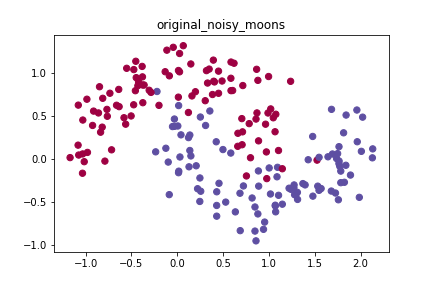# K Nearest Neighbor(KNN)

KNN顧名思義就是找K個最近的點，然後讓他們投票，如果與你最近的五個資料點當中，有3個是第一類，那麼這個測試點就是第一類。這個做法非常簡單、暴力，因此也常常被當作baseline基準(也就是最陽春的做法，其他作法一定要比這樣的做法又更好的表現才有意義)。

``````for name, X, Y in datas:
clf = KNeighborsClassifier(n_neighbors=3)  ## 設定用最近的3個鄰居投票
clf.fit(X, Y)  ## 訓練模型

y_pred = clf.predict(X)  ## 預測模型
print('Accuracy',  str((Y == y_pred).sum()/ X.shape*100)+"%")  ## 計算精準度

plot_decision_boundary(lambda x: clf.predict(x), X.T, Y)  ## 視覺化分類器的分類結果
plt.savefig(os.path.join('pic', name+'_knn'))  ## 儲存圖片
plt.show()
``````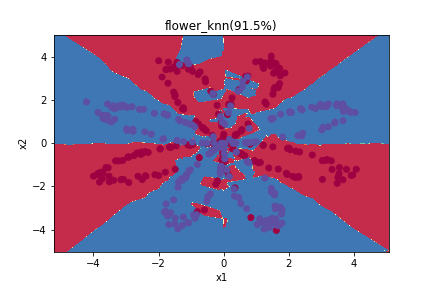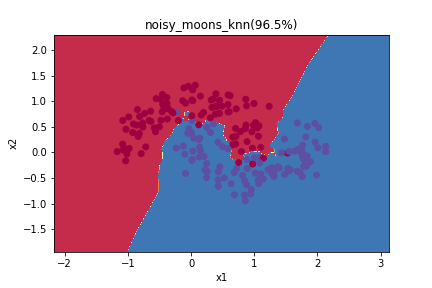# Logistic Regression``````for name, X, Y in datas:
clf = LogisticRegressionCV()
clf.fit(X, Y)

y_pred = clf.predict(X)
print('Accuracy',  str((Y == y_pred).sum()/ X.shape*100)+"%")

plot_decision_boundary(lambda x: clf.predict(x), X.T, Y)
plt.title(name+'_logistic(' + str((Y == y_pred).sum()/ X.shape*100)+"%)")
plt.savefig(os.path.join('pic', name+'_logistic'))
plt.show()
``````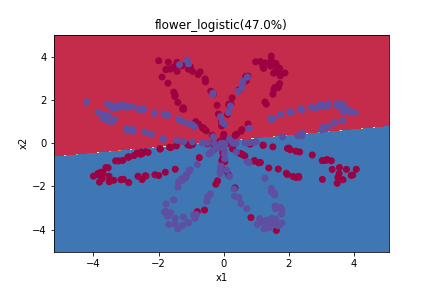# Decision Tree

<=30 high no fair no
<=30 high no excellent no
30…40 high no fair yes
>40 medium no fair yes
>40 low yes fair yes
>40 low yes excellent no
31…40 low yes excellent yes
<=30 medium no fair no
<=30 low yes fair yes
>40 medium yes fair yes
<=30 medium yes excellent yes
31…40 medium no excellent yes
31…40 high yes fair yes
>40 medium no excellent no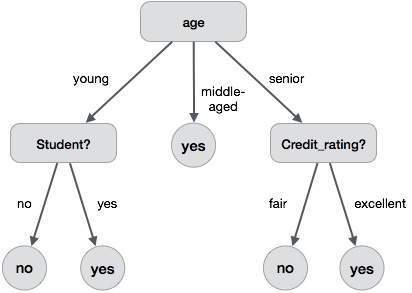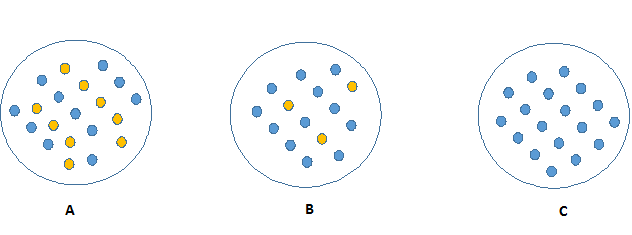``````for name, X, Y in datas:
clf = DecisionTreeClassifier()
clf.fit(X, Y)

y_pred = clf.predict(X)
print('Accuracy',  str((Y == y_pred).sum()/ X.shape*100)+"%")

plot_decision_boundary(lambda x: clf.predict(x), X.T, Y)
plt.title(name+'_tree(' + str((Y == y_pred).sum()/ X.shape*100)+"%)")
plt.savefig(os.path.join('pic', name+'_tree'))
plt.show()
``````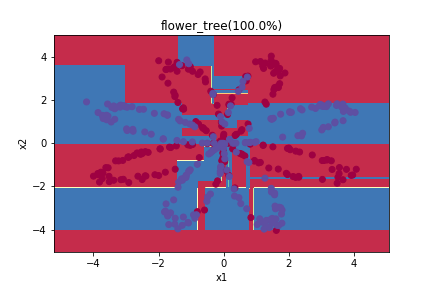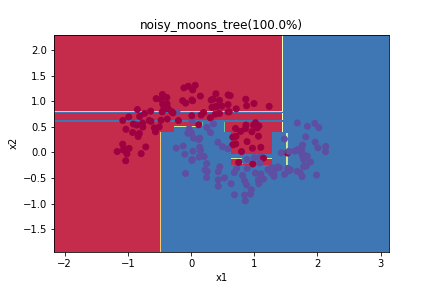code在這裡